# Benchmark of Fugue on Spark#

As an abstraction layer, how much performance overhead does Fugue add to native Spark?

In the original article, the author presented this performance comparison chart: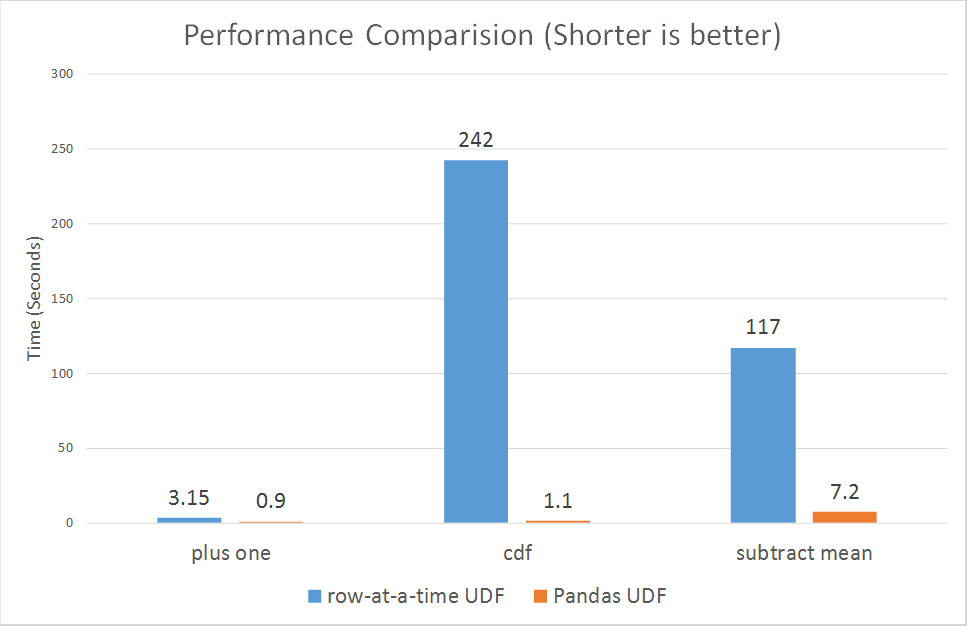In this article, we will keep using the same examples and same datasets. We will compare the performance of row-at-a-time-UDF, Fugue on Native Spark, Pandas UDF, Fugue on Pandas UDF, and Fugue SQL. We will also test on additional datasets to provide a better picture of the performance difference.

Notice that to make Fugue run on native Spark or Pandas UDF, you only need to toggle the configuration fugue.spark.use_pandas_udf (see details).

The associated notebook can be found here or here.

## Configuration#

• Databricks runtime version: 10.1 (Scala 2.12 Spark 3.2..0)

• Cluster: 1 i3.xlarge driver instance and 8 i3.xlarge worker instances

## The Datasets#

The original example dataset is a Spark dataframe with 10 million rows, it was created and persisted in this way:

df = spark.range(0, 10 * 1000 * 1000).withColumn('id', (col('id') / 10000).cast('integer')).withColumn('v', rand())
df.cache()
df.count()


We used the same approach to create df_larger with 40 million rows, and df_largest with 200 million rows. We also created df_more_parts with 20000 partitions:

df_larger = spark.range(0, 40 * 1000 * 1000).withColumn('id', (col('id') / 10000).cast('integer')).withColumn('v', rand())
df_larger.cache()
df_larger.count()

df_largest = spark.range(0, 200 * 1000 * 1000).withColumn('id', (col('id') / 10000).cast('integer')).withColumn('v', rand())
df_largest.cache()
df_largest.count()

df_more_parts = spark.range(0, 10 * 1000 * 1000).withColumn('id', (col('id') / 5000).cast('integer')).withColumn('v', rand())
df.cache()
df.count()


## Cumulative Probability#

This is a simple map problem that can’t be replaced with standard SQL. For df and df_larger we tested on Native Spark, Fugue on Native Spark, Pandas UDF and Fugue on Pandas UDF. For df_largest we excluded the Native Spark approach because it took too long.

For Fugue, we rewrite the logic as a simple Python function

def fugue_cdf(df:pd.DataFrame) -> pd.DataFrame:
return df.assign(v=stats.norm.cdf(df.v))


Here is the performance chart: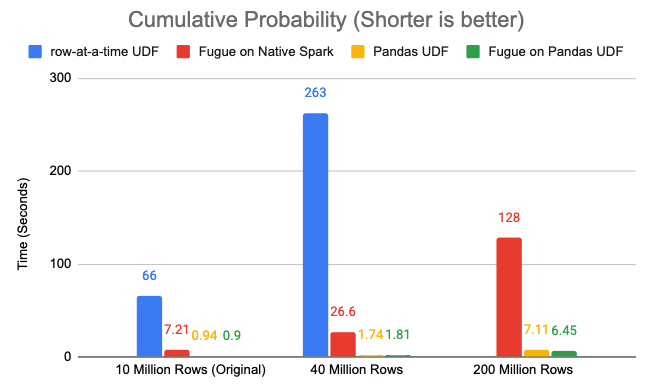• Fugue on Native Spark is roughly 9x to 10x faster than row-at-a-time UDF.

• With Pandas UDF, the overhead of Fugue is less than 0.1 seconds regardless of data size.

• Pandas UDF is indeed much faster.

The reason Fugue on Native Spark is so much faster is that we have done non-trivial performance optimization on native Spark wrapping logic. The input and output data types of the python functions will also affect the speed, both the data conversion and the compute inside the function will be factors. In this particular case, the data conversion (from Spark Rows to Pandas dataframe without Pandas UDF) will add overhead, but the compute using Pandas will reduce overhead, and when they add together, it turns out to be faster, but this doesn’t apply to general cases.

We must also notice that a distributed system’s performance is not as stable as a local system. Even if you see in some cases using Fugue on Pandas UDF are faster than Pandas UDF itself, it’s mainly because of the performance variance. What we want to show here is that performance-wise, Fugue on Pandas UDF is on the same level as Pandas UDF, there isn’t obvious overhead.

## Subtract Mean#

The author’s approach is groupby-apply, which is a valid idea to compare the performance. And to examine the performance overhead brought by Fugue, we will also consider 3 more scenarios here:

• With longer compute: each partition processing logic can take longer, so we let it sleep 0.5 seconds before returning the result.

• With more partitions: if there is overhead to process each partition, then increasing the number of partitions could increase the performance difference. Here we will use df_more_parts.

• With more data in each partition: to serve the same purpose as the previous one but from a different perspective. Here we will use df_larger

For Fugue, we rewrite the logic as a simple Python function:

def pandas_subtract_mean(pdf:pd.DataFrame, sec=0.0) -> pd.DataFrame:
sleep(sec)
return pdf.assign(v=pdf.v - pdf.v.mean())


Here is the performance chart: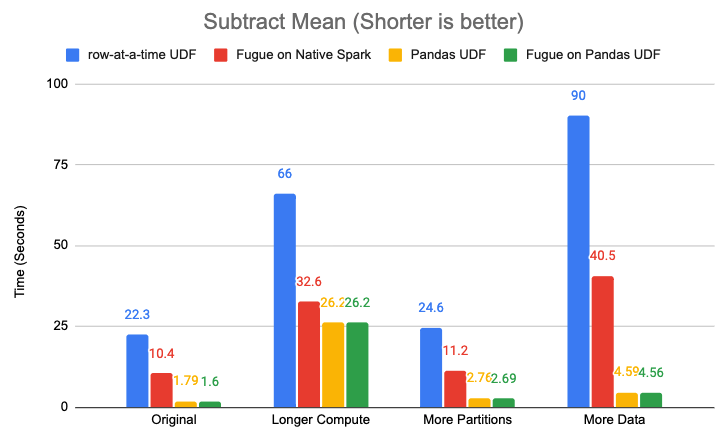• Fugue on Native Spark is over 2x faster than row-at-a-time UDF.

• With Pandas UDF, the overhead of Fugue is not obvious, regardless of data size, compute size, or partition size.

• Pandas UDF is indeed much faster, but with longer compute, Pandas UDF has less speed advantage.

• With the same amount of data, more partitions always require more time to process.

But if we rethink this scenario. This can be done by calculating the average of each id and joining back to compute the difference. It is a typical case where SQL can provide an elegant solution, here is the Fugue SQL solution:

m = SELECT id, AVG(v) AS mean FROM df GROUP BY id
SELECT df.id, df.v-mean AS v FROM df INNER JOIN m ON df.id = m.id
SELECT SUM(v) AS s
PRINT


Fugue SQL on Spark will be translated to a sequence of Spark SQLs to run. We tested on df, df_larger and df_largest: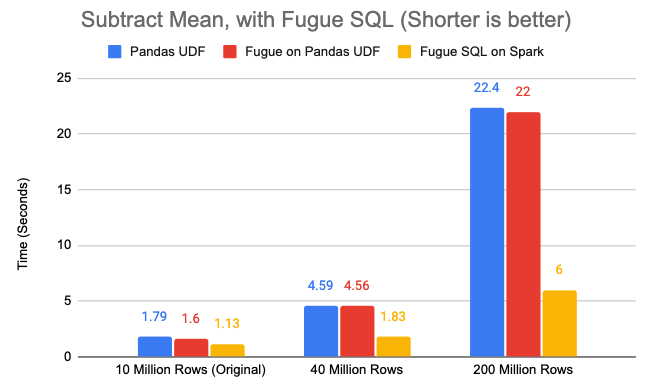So the SQL approach is always the fastest regardless of data size. This is an important rule of thumb: try to keep Spark compute in JVM.

## Plus One#

The reason we put this example the last is because it is not a good example as the Cumulative Probability. Based on the previous examples, we can tell the Pandas UDF will beat the one-row-at-a-time UDF again. But how does Pandas UDF compare to a SQL solution? Since we have verified the overhead of Fugue on Pandas UDF in the previous examples, in this example, we will just compare Pandas UDF and Fugue SQL:

SELECT id, v+1 AS v FROM df
SELECT SUM(v) AS v
PRINT


Again we tested on df, df_larger and df_largest: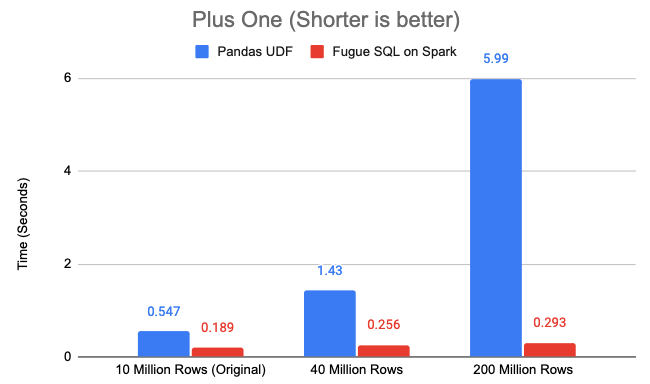It simply reiterates the rule of thumb: try to keep Spark compute in JVM. Why SQL is important for Spark? One of the reasons is that it naturally keeps most of the logic inside JVM, so Spark can run efficiently.

## Conclusion#

Considering the 3 examples above:

• Without Pandas UDF, Fugue is highly optimized to run on PySpark. It usually outperforms simple native PySpark code such as row-at-a-time UDF

• With Pandas UDF, Fugue doesn’t have a noticeable overhead against the native Pandas UDF approach, regardless of data size, compute size, and partition size. (Based on our other tests, Fugue on Pandas UDF may have a fixed overhead on a sub-second level regardless of data size.)

• Fugue SQL on Spark can easily outperform Pandas UDF if the logic can be expressed in SQL.

The core value of Fugue is to make distributed computing easy and consistent so every user can fully leverage the computational power they have. Our focus is optimizing real use cases on simplicity, testability and logical independence. We are not interested in any benchmark competitions and we do not attempt to beat the underlying computing frameworks on performance. In practice, it’s common to see a certain level of performance overhead due to the nature of wrapping logic. But we have been working hard to minimize the overhead when delivering our core value to users.